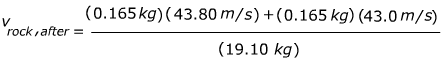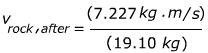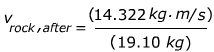# Momentum Formula

Momentum Formula

Momentum is a quantity with a value and a direction. It is the product of the mass of an object and its velocity. Momentum is conserved in elastic collisions. The unit of momentum is a kg·m/s, which is also equivalent to a J·s (a Joule·second).

momentum = (mass)(velocity)

p = mv

p = momentum (kg·m/s)

m = mass (kg)

v = velocity (m/s)

Momentum Formula Questions:

1) The combined mass of a motorcycle and the person riding it is 200.0 kg. If the rider is traveling at a constant velocity of 30.0 m/s, what is the momentum of the motorcycle and rider?

Answer: The momentum can be found using the formula:

p = mv

p = (200.0 kg)(30.0 m/s)

p = 6000 kg·m/s

The momentum of the motorcycle and rider is 6000 kg·m/s.

2) A hockey puck is sliding across the ice at a velocity of 43.80 m/s. It has a mass of 0.165 kg. The puck hits a 19.10 kg curling rock that is initially at rest. The hockey puck bounces off the rock, and slides in the opposite direction at 43.0 m/s. What is the velocity of the curling rock after the collision?

Answer: This question depends on the conservation of momentum in elastic collisions. The total momentum before is equal to the total momentum after:

ppuck,before + prock,before = ppuck,after + prock,after

mpuck vpuck,before + mrock vrock,before = mpuck vpuck,after + mrock vrock,after

The mass of the puck is mpuck = 0.165 kg. The mass of the rock is mrock = 19.10 kg. The velocity of the puck before the collision is vpuck,before = +43.80 m/s. The velocity of the rock before the collision is vrock,before = 0.0 m/s, because it was at rest. The velocity of the puck after the collision is negative, because it was moving in the opposite direction as before the collision: vpuck,after = -43.0 m/s.

(0.165 kg)(43.80 m/s) + (19.10 kg)(0.0 m/s) = (0.165 kg)(-43.0 m/s) + (19.10 kg)vrock,after

(0.165 kg)(43.80 m/s) = -(0.165 kg)(43.0 m/s) + (19.10 kg)vrock,after

Now, the equation can be rearranged to find vrock,after.

(0.165 kg)(43.80 m/s) + (0.165 kg)(43.0 m/s) = (19.10 kg)vrock,aftervrock,after = +0.750 m/s

The velocity of the curling rock after the collision with the hockey puck is density of ice is vrock,after = +0.750 m/s.

 Related Links: Momentum and Impulse Quiz Momentum Quiz Momentum Quiz Momentum Examples Momentum and Impulse Examples